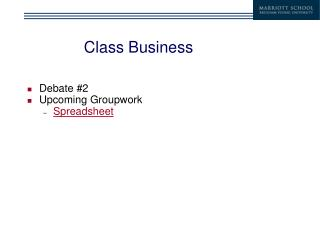DownloadDownload PresentationDownload Presentation- - - - - - - - - - - - - - - - - - - - - - - - - - - E N D - - - - - - - - - - - - - - - - - - - - - - - - - - -
##### Presentation Transcript

1. Class Business • Debate #2 • Upcoming Groupwork • Spreadsheet

2. Covariance: From Probability model • Cov[r1,r2] =E[(r1-E[r1]) (r2 -E[r2])] Steps: • Find expected return for each asset • Find deviations from mean for each asset in each state. • Take product of deviations • Find expected product of deviations

3. Covariance: Probability Model • Step 1: Find expected return for each asset E[r1]=.80(.10) + .20(-.05) = 0.07 E[r2]=.80(.05) + .20(0.0) =0.04 • Step 2: Find Deviations

4. Covariance: Probability Model • Step 3: Find product of deviations in each state • Product1=.03(.01)=.0003 • Product2= (-.12)(-.04)= .0048 • Step 4: Find expected product of deviations • Covariance = .8(.0003) +.2(.0048)=.0012

5. Covariance: Probability Model • Even if we don’t know correct probability model, we can still estimate the covariance from past data. Average Covariance is average product of deviations.

6. Correlation • Correlation is defined as:

7. Correlation and Variance • Suppose • E[rA]=8%, sA=0.10 • E[rB]=12%, sB=0.17 • Simulation

8. Correlation and Variance • Create portfolios of stock A and B

9. Correlation and Variance • The correlation between A and B is -1. • When the correlation is -1, it is always possible to find a portfolio with zero variance. • In general, the variance of a portfolio with two assets is given by

10. Portfolio Allocation: Two Risky Assets • If the correlation is -1 between two risky assets, you can always create a risk-less portfolio by combining the two. • It may not be desirable to do this. Why? • Risk-free assets earn only the risk-free rate. • Depending on your level of risk aversion, you may want to take on more risk and get a higher expected return.

11. Portfolio Allocation: Two Risky Assets • How do you decide how to allocate your investment equity between two risky assets? • We’ll first consider the case in which -1< r < 1

12. Portfolio Allocation: Two Risky Assets • E[r1]=.20, s1=.20 • E[r2]=.325, s2=.25 • rf=.10 Why ever invest in asset 1? Asset 2 Asset 1

13. Portfolio Allocation: Two Risky Assets • Why ever invest in asset 1? • What is the trade-off between risk and return using two risky assets? • Assume Cov[r1,r2]=.025 • (This implies )

14. Portfolio Allocation • Suppose we form a portfolio with just these two risky assets. • How is expected return a function of standard deviation? • Messy Algebra (e.g. see Ingersoll, “Theory of Financial Decision Making”, chapter 4).

15. Portfolio Allocation • Instead of doing algebra, let’s just make a plot in Excel. • Step one: specify various portfolio weights • Step two: calculate E[rp], Stdev[rp] • Step three: plot values • Excel Spreadsheet

16. Portfolio Allocation • What does the graph look like? “Efficient Frontier” Asset 2 Asset 1 minimum variance portfolio

17. Portfolio Allocation • How does this help us make portfolio allocation decisions? • Can pick combination of “risk” and “return” that you want, and back out portfolio weights. Suppose you want E[rp] = 25% Then you must take std. dev = .186 .

18. Portfolio Allocation • But we know that • So • Just solve for w and you have made your portfolio allocation decision.

19. Portfolio Allocation • In general, investors prefer points to the “northwest”, since these points earn higher expected returns for lower risk. Clearly, the minimum variance portfolio is preferred to asset 1. What about asset k? Minimum variance portfolio Asset k Asset 1

20. Portfolio Allocation • To choose optimal mix of two risky assets, first consider adding in risk-free asset to our portfolio. Asset 2 T Asset 1

21. Portfolio Allocation: Two Risky Assets • When -1<r<1 between two risky assets, it is always possible to find a portfolio of these two assets whose CAL is steeper than the CAL of either of the two original assets. • When r= 1 or -1 the efficient frontier is composed of straight lines • (see p. 180 in text)